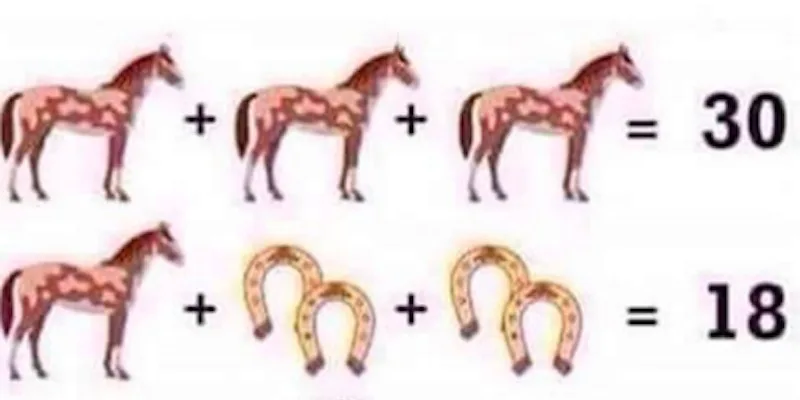News# This Simple Math Question Has Everyone On The Internet Completely Stumped

The fact that there are horse pictures has to mean this math question is easy, right? Wrong.

This question is winding up the world wide web. Answers vary wildly.

Just the thought of algebra is stressful enough to make me want to slam my computer. But surprisingly, a lot of people have been giving the question a try.

We've all resorted to some pretty low blows in our anger. One user on Imgur accused another of "reducing the gene pool," and said "it would be helpful if you do not breed much."

Ouch. Get some cream for that burn.

### Let's see how we got there:

• The horse is X.
• The horseshoe is Y.
• The boot is Z.

3X = 30

X=10

### Finding Y:

First trick: The math equation counts two horseshoes in one.

Make sure you count them as two because in the last equation, there is only one.

10 + Y + Y + Y + Y = 18

10 + 4Y = 18

4Y = 8

Y = 2

### Finding X:

4-2Z=2

Second trick: Most of the answers on the internet are wrong because they have confused the order in which to complete the equation, which is a system referred to as BODMAS.

The correct way to lay out the equation is 1+(10x2). NOT 1+10x2.

The answer is 21, as 10 x 2 = 20, and 20 + 1 = 21.

You're welcome.